# Measurement and Analysis of Thermal-Hydraulic Performance of Curved and Plate Flat Solar Air Heaters; A Comparative Study

Measurement and Analysis of Thermal-Hydraulic Performance of Curved and Plate Flat Solar Air Heaters; A Comparative Study
Laboratoire de Génie Mécanique, Université Mohamed Khider B.P. 145, Biskra, Algérie

Laboratoire de Génie Mécanique, Université Mohamed Khider B.P. 145, Biskra, Algérie

Corresponding Author Email:
a.labed@univ-biskra.dz
Page:
553-558
|
DOI:
https://doi.org/10.18280/i2m.180606
9 June 2019
|
Accepted:
12 October 2019
|
Published:
30 December 2019
| Citation

OPEN ACCESS

Abstract:

This paper presents a comparative study between the thermal-hydraulic performances of two types of solar air heaters (SAHs) having the same components: i) is a simple pass solar flat plate collector, and ii) is a simple pass curvilinear solar collector. Thus, we carried out studies to compare the thermal efficiencies and hydraulic performances of both SAHs, in order to compare their effective efficiencies. The experimental measures show that, at different air mass flow rates, the highest efficiencies were obtained from the curvilinear SAH and no significant difference between the pressure drops in both SAHs is noticed. In addition, this study has allowed us to show that the curvilinear form is an efficient method to improve the SAH performances, it permits a good temperature distribution in the air flow duct of the collector.

Keywords:

solar collector, air heater, curved collector, efficiency, thermal-hydraulic

1. Introduction

The transformation of solar radiation into thermal energy is based on systems known as solar collectors. This energy is used directly by relying on so-called passive systems by storing it in building elements (conservatory, greenhouse, glass facade) or indirectly through so-called active solar collector systems via heat transfer fluid which can be water or air. They are used for heating buildings and for drying food products and agri-food products [1-3].

Solar collectors have generally flat form. These collectors can be improved by the addition of baffles (obstacles) in the flow channel of the caloporting fluid .

For this purpose, thermal improvement was proposed by acting on the flow direction of the heat transfer fluid by Labed et al. . The system has been tested under natural conditions for different flow rates of the caloporting fluid. They found that efficiency increases with the increase in flow rate; in addition, the thermal efficiency of Solar air heater is higher when the air flow is oriented from the high entrance to the low exhaust.

Several attempts have been carried out to investigate thermo-hydraulic performances of roughened solar air heater numerically [6, 7] and experimentally [8-10]. They proved that roughened solar air heaters are quantitatively and qualitatively better than conventional solar air heater. Various parameters were studied such as mass flow rate, the number of the glass cover, variation in the width of the duct, roughness geometries and air velocity, etc. These parameters affect thermal and effective efficiencies of roughened solar air heater.

Another variant has been developed, characterized by the curvilinear shape by Mahboub et al. . They designed, constructed and experimented a new SAH model, characterized by the curvilinear shape. The designed model has the same materials and dimensions as those of a conventional system (plate SAH). It has been concluded that the proposed design has advantages not only in terms of thermo-hydraulic performance, but also, this new design does not require the use of artificial roughness unlike any other efficient insolator and therefore, the cost of manufacture and the weight are minimized.

Two years later; Singh et al.  investigated numerically various curved solar air heater designs that shows signiﬁcant enhancement of heat transfer and thermal efficiency. They have taken the initial design proposed in the reference . The Computational Fluid Dynamic (CFD) model is validated by the results reported by Mahboub et al. . They observed that secondary vortex formation near the absorber wall increases the Nusselt number signiﬁcantly. New correlations for friction factor and Nusselt number has been developed as a function of Reynolds number and various geometric parameters such as relative groove height.

Furthermore, Singh et al.  investigated experimentally a curved SAH using a validated computational fluid dynamic model for different curvature angles (25–50°). In order to enhance thermo-hydraulic performance by incorporating chimney effect on convex and concave flow channel. The results show significant heat transfer enhancement factor, increase in Nusselt number per pressure drop unit (NuP) and temperature enhancement ratio in comparison to conventional flat SAH. The convex and concave designs present respectively, a thermal enhancement of 43% and 31% and NuP of 7% and 6% higher than conventional flat natural convection SAH.

Recently Singh et al.  presented a comparison of the thermal performances of a curved and flat solar air heater (SAH) under diverse environmental conditions using the above cited numerical model. They determined the optimum curvature angle for curved SAH under wide range of mass flow rate (0.0172–0.0472 kg/sm2) that offers optimum thermal performance (i.e. 25°), Reynolds numbers (2200-6000) and inclination angle (0–60°). The enhancement factor (Nucurved/Nuflat) is in the range 1.5-2.2 which show that curved SAH is thermally much better than flat design. Secondly, the optimized curved SAH is then compared with flat design for 0–60° tilt angles θ, under tranquil and windy conditions.

In this work, we propose to make a comparative study between the thermal performances of the flat and curvilinear SAHs. Furthermore, the hydraulic performances such as the pressure drop and the electrical power consumption were measured. The main objective was to determine the effective performances of both SAHs. Generally, efficiency was the most important factor in predicting the thermal and effective performances of solar air collectors.

2. Experimental

2.1 Experimental setup

The conducted experimental setup consists of two similar air solar collectors, flat and curvilinear (Figure 1) whose technical characteristics are:

-   The transparent cover is made of Plexiglas with a thickness of 3 mm.

-   The absorber is a galvanized steel sheet with a thickness of 1 mm and 0.8 m × 1.6 m of surface painted in matte black.

-   The insulation is made of polystyrene with a thickness of 4 cm.

-   Useful duct length L = 1.6 m.

-   Width of the useful duct l = 0.8 m.

-   The inclination of the insolator i = 34 °.

-   The flow is below the absorber.

-   The distance between the absorber and the transparent cover is equal to 2.2 cm (Figure 2).

## 1.png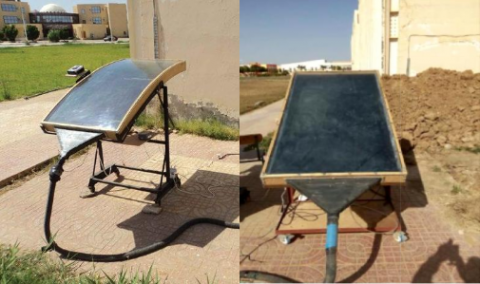(a)                                                           (b)

Figure 1. Experimental devices: a) plate SAH, b) curved SAH

## 3.png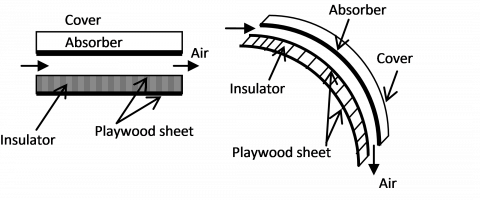Figure 2. Schematic view of both solar collectors

2.2 Experimental measurements

The experiments were carried out in an open air near the technological hall of the Department of Mechanical Engineering of Biskra University (Latitude: 34° 50'43.65'' N, Longitude: 5° 44'49.27'' E, Altitude: 107 m). Measurements are made during sunny days with sometimes cloudy periods. The collectors were placed in a stand facing south at an inclination angle equal to the local latitude.

In both studied collectors, the air outlet cross-sections are equipped with divergent channel duct, the test facility permits to vary the mass flow rate of the air. In summary, the above experimental set-up is used for the measurement of the solar radiation, wind velocity, pressure drop and the air temperatures in the inlet, outlet, absorber plate surface and the air channel duct.

To carry out these experiments, we chose to use twelve (12) points of measurement; ten (10) insulated thermocouples have been used for the mean temperature measurement in the channel duct of the SAHs, outlet air temperature is measured by a thermocouple and the ambient temperature is measured by a mercury thermometer.

The air temperatures readings are based on a simple mesh, each temperature value is measured at half height point (z=e/2), four (04) intervals following the flow direction axis (x) (small mesh sections at the entrance and exit, and wide sections in the middle of the collector) and two (02) intervals on the half-width of air channel duct perpendicular to the flow direction (y) (by considering that the air temperature is symmetric). The temperature values are recorded according to the points shown in the following illustration (Figure 3).

## 3a.png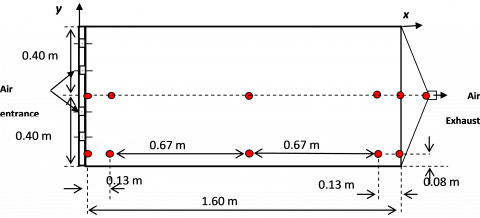Figure 3. Points of measurement of the temperatures in the air flow channel duct

The test data were measured at an average interval of 30 min, the temperatures, solar radiation intensity, pressure drop, the air flow rate and wind velocity are, respectively, measured with the k-type thermocouples with an accuracy of 0.01℃, Kipp and Zonen pyranometer CM11 with 1%accuracy, pressure transducer accuracy (Kimo CP301) with ±1 Pa and 0.5% of reading, a Kimo-type anemometer with hot wire (VT300) with ±3% of reading and ±10m3 for the flow rate measurement and ±3% of reading and ±0.1m/s accuracy were used.

2.3 Experimental uncertainty analysis

The performance of a solar collector can be evaluated from the following global energy balance that indicates the distribution of received solar energy by the SAH into useful energy gain $Q_{u},$ thermal losses $Q_{p},$ and stored energy $Q_{s t}$

$Q_{s a}=Q_{u}+Q_{p}+Q_{s t}$     (1)

By neglecting the thermal energy stored in the various components of the collector (thermal inertia), we obtain:

$Q_{s a}=Q_{u}+Q_{p}$          (2)

The quantity of energy necessary for the heating of the caloporting fluid can be expressed by:

$Q_{u}=\dot{m} . C_{p} .\left(T_{o u t}-T_{i n}\right)$      (3)

The solar collector efficiency, defined as the ratio between the amounts of useful energy recovered and the incident global radiation is written as follows:

$\eta_{t h}=\frac{Q_{u}}{I \times S_{a b s}}$            (4)

Also translated by:

$\eta_{t h}=\dot{\mathrm{m}} . C_{p} \frac{\left(T_{o u t}-T_{i n}\right)}{I \times S_{a b s}}$        (5)

where, $C \mathrm{P}$ is the specific heat of the air, $S_{a b s}$ is the active area of the absorber.

The effective efficiency is function of useful energy gain and the measured fan power consumption $(P m)$ given in Eq.(6) [ 15]:

$\eta_{e f f}=\frac{Q_{u}-P m}{I \times S_{a b s}}$         (6)

Based on the analysis of the errors in the experimental measurements through the use of instruments, the uncertainties in experimental measurement and results are often used to refer to possible values that may include errors. the result $R$ of an experiment is assumed to be calculated from a set of measurements, it is given as a function of the independent variables $X 1, X 2, \ldots, \operatorname{Xn}$

$\mathrm{R}=\mathrm{R}(X 1, X 2, X 3, \ldots, X n)$              (7)

where, X1, X2, X3 are the measured variables.

Let $\delta R$ be the uncertainty in the result and $\delta X 1, \delta X 2, \ldots$ $\delta X n$ be the uncertainties in the independent variables. If the uncertainties in the independent variables have the same odds, then uncertainty in the result having these odds is calculated by the following equation [ 14]:

$\delta R=\left[\left(\frac{\partial R}{\partial x_{1}} \delta X 1\right)^{2}+\left(\frac{\partial R}{\partial x_{2}} \delta X 2\right)^{2}+\ldots+\left(\frac{\partial R}{\partial x n} \delta X n\right)^{2}\right]^{1 / 2}$  (8)

The independent parameters measured in the experiments reported here are: collector inlet temperature Tin, collector outlet temperature Tout , ambient temperature Ta, mass flow rate and solar irradiation.

If $A$ and $C_{p}$ are considered constants in Eq. $(3),$ it can be written as:

$\eta=f(\text { Tout }, \operatorname{Tin}, I, m)$    (9)

The total uncertainty equation for collector efficiency, can be written as:

$\delta \eta=\left[\left(\frac{\partial_{\eta}}{\partial m} \delta m\right)^{2}+\left(\frac{\partial_{\eta}}{\partial \text { Tout }} \delta \text { Tout }\right)^{2}+\left(\frac{\partial \eta}{\partial \operatorname{Tin}} \delta \operatorname{Tin}\right)^{2}+\left(\frac{\partial n}{\partial 1} \delta I\right)^{2}\right]^{1 / 2}$     (10)

Calculations show that the total uncertainty in calculating efficiency $\eta$ are almost in the order of 1%.

3. Results and Discussion

The evolution of the different parameters is measured for both SAHs simultaneously, every 30 minutes from 09:00 am to 16:00 pm. Figures 4 and 5 show the profiles of the measured global solar radiation intensity and the difference in air temperature between inlet and outlet for the experimental days of 27/02/2018 and 17/04/2018, respectively. The examination of the curves of these tow figures shows that, this difference strongly depends on the global solar radiation. This difference is also, more significant around solar noon.

## 4.pngFigure 4. Difference in air temperature (outlet-inlet) and solar intensity (27/02/2018), m = 0.0253 kg/s

Figures 6 and 7 show the evolution of the global solar radiation and the thermal efficiency of both collector for the same days (27/02/2018 and 17/04/2018) and for the same air flow rates (0.0253 and 0.0204 Kg/s), respectively. It can be noted that, and under the same conditions, the curved SAH presents the highest thermal efficiency. This is may be due to the effect of the curvilinear geometry of the absorber plate which makes more turbulence for the air particles. The examination of these curves shows that, the thermal efficiency depends strongly on the global solar radiation. Furthermore, the difference between the performances of both SAHs is more significant around solar noon.

## 5.png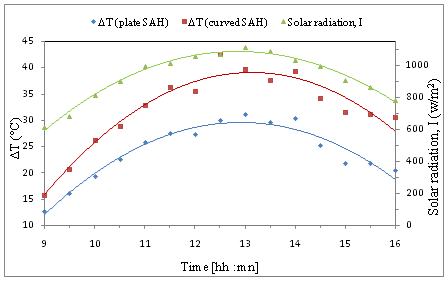Figure 5. Difference in air temperature (outlet-inlet) and solar intensity (27/02/2018), m= 0,0204 kg/s

## 6.pngFigure 6. Thermal efficiency and intensity of solar radiation during the experimental days (27/02/2018), m = 0.0253 kg/s

## 7.png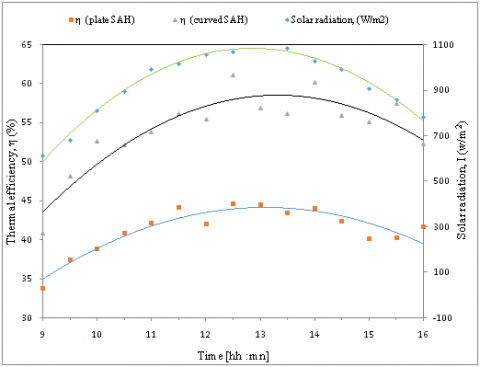Figure 7. Thermal efficiency and intensity of solar radiation during the experimental days (17/04/2018), m = 0.0204 kg/s

The curves in Figure 8 indicate the variation of efficiency as a function of mass flow for a typical day. It should be noted that the thermal efficiency increases with the increase in the flow rate value.

We note also that the thermal efficiency of the curved SAH is higher than that of the plate SAH, a significant difference between the efficiency values of both SAH models is recorded from a flow rate value equal to 0.015 kg/s.

The temperature distribution is obtained by the reading in the experiment points presented in Figure 3. The Figures 9 and 10 show the contours of the temperature distribution of the air in the channel ducts of both SAH models; the plate SAH and the curved SAH, successively, for air flow rate equal to 0.033Kg/s and for solar intensity I equal to 850 W/m2. It can be seen that the temperature distribution is not the same, neither at the entrance or the middle, nor at the outlet of the channel duct of both SAHs.

The distribution of air temperature along the solar air heater channel shows an increase tendency from the entrance to the exhaust. The maximum values of temperature are obtained in the exhaust of the collector for both SAHs. By comparing between the temperature contours of both SAHs (Figures 9 and 10). It can be noted that, the air temperature values along the channel duct of the curved SAH are higher than that of the plate SAH.

The values of the temperature measured in the middle line e/2 of both SAHs are higher than those near the side walls; this may be due to the heat losses through the side walls.

## 8.png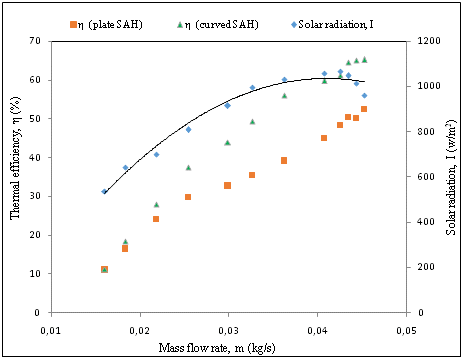Figure 8. Variation of the thermal efficiency Vs air mass flow rate, (10/4/2018)

## 9.png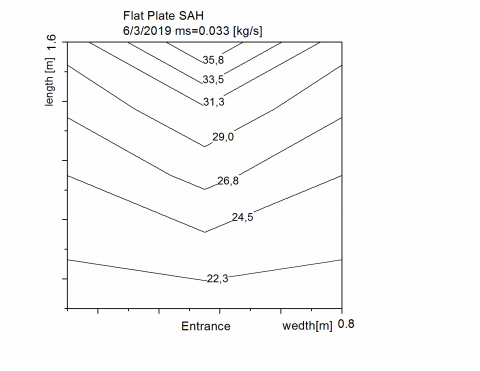Figure 9. Air temperature distribution in the flow channel duct of the plate SAH

## 10.png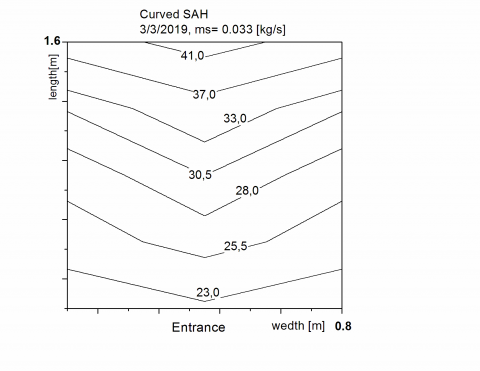Figure 10. Air temperature distribution in the flow channel duct of the curved SAH

Figure 11 give an idea about the pressure drop between the entrance and the exhaust of both SAHs for different air flow rates. It can be noted from the figure that the pressure drop in the curved SAH is higher than that of the plate SAH, whereas this difference is not significant in comparison with the gain in the thermal performances. However, a higher mass flow rate also results in higher friction factor values

## 11.png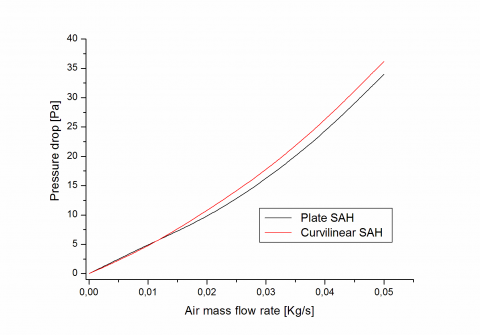Figure 11. Pressure drop Vs air flow rate for SAHs models

The variation of the thermal efficiency average (η) is expressed as a function of the variation in the air flow rate. It should be noticed that the thermal efficiency increased considerably with increasing air flow rate, its curve increases as a convex pace. The same evolution occurred for the electrical consumption values in Figure 12, we note that the measured fan power consumption for both SAHs increases gradually with increasing air flow rate; however, its curve increases as a concave pace.

Therefore, the pumping power required is converted to equipment thermal energy, in order to evaluate the real performance of the collector, in terms of the effective efficiency for the useful thermal gain, and the equivalent thermal energy that will be required to provide corresponding mechanical energy for overcoming friction power losses, this directly affect the effectiveness efficiency which increases with increasing in the air flow rate as straight line curve (Figure 13).

## 12.pngFigure 12. Fan electrical consumption Vs air flow rate for both SAHs models

## 13.png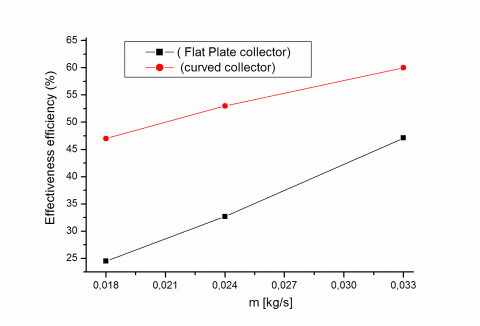Figure 13. Effectiveness efficiencies Vs air flow rate for both SAHs models

4. Conclusion

From the comparative study conducted between the plate and curvilinear solar air heaters (SAHs), an investigation of their thermal-hydraulic performances is provided. Their performances are very sensitive to various climatic parameters, especially the intensity of the solar radiation.

It is noted that, under the same conditions, the curved SAH presents the more efficient model. This is may be due to the effect of the curvilinear geometry of the absorber plate which creates more turbulence for the air particles. This superiority of the curved SAH is more significant at solar noon

The air temperature contours in the channel ducts of both SAHs indicate that the air temperature values along the channel duct of the curved SAH are higher than that of the plate SAH. The maximum temperature values are obtained in the exhaust of the collector for both SAHs. The temperature values in the medium line of both SAHs are always higher than those of the sides.

The pressure drop in the curved SAH is higher than that of the plate SAH, whereas this difference is not significant in comparison with the gain in the thermal performances.

Acknowledgment

The authors want to express their acknowledgment and gratitude to Mr. Youcef Bekkari and Mr. Rizq Abu-Hasnah (ex-master students at the University of Biskra) for their technical assistance in the preparation of the experimental setup.

Nomenclature
 I ṁ Solar radiation, W.m-2 Mass flow rate of the air, kg.s-1 CP De DH F0 Specific heat of the air, J. kg-1. K-1 Dean number Hydraulic diameter, m Heat removal factor based on air outlet temperature Pm Qsa Qu Qp Qsk  UL Re RC Sabs Ta Tin Tout Vv Electrical power consumption, W Amount of heat absorbed, W.m-2 Amount of useful heat, W.m-2 Amount of heat lost, W.m-2 Amount of stored heat, W.m-2 Overall loss coefficient, W.m-2.K-1 Reynolds number Curvature radius, (m) Surface of the absorber, m2 Ambient temperature, °C Air inlet temperature, °C Air outlet temperature, °C Wind speed, m.s-1 Greek symbols $\eta_{t h}$ Thermal efficiency (%) τ Transmittance a Absorptance
References

 Kuma, V., Prasad, L. (2018). Performance prediction of three sides hemispherical dimple roughened solar duct. Instrumentation Mesure Métrologie, 17(2): 273-293. https://doi.org/10.3166/I2M.17.273-293

 Labed, A., Moummi, N., Aoues, K., Benchabane, A. (2016). Solar drying of henna (Lawsonia inermis) using different models of solar flat plate collectors: An experimental investigation in the region of Biskra (Algeria). Journal of Cleaner Production, 112: 2545-2552. https://doi.org/10.1016/j.jclepro.2015.10.058

 Murmu, R., Kumar, P., Singh, H.N. (2018). Experimental investigation on heat transfer and friction factor for an inclined spherical ball roughened solar air heater. Instrumentation Mesure Métrologie, 17(1): 7-36. https://doi.org/10.3166/I2M.17.7-36

 Blaise, K.K., Magloire, K.E.P., Prosper, G. (2018). Thermal performance evaluation of an indirect solar dryer. Instrumentation Mesure Métrologie, 17(1): 131-151. https://doi.org/10.3166/I2M.17.131-151

 Labed, A., Moummi, N., Benchabane, A., Zellouf, M. (2015). Experimental analysis of heat transfer in the flow channel duct of solar air heaters (SAHs). International Journal of Heat and Technology, 33(3): 97-102. http://dx.doi.org/10.18280/ijht.330314

 Gawande, V.B., Dhoble, A.S., Zodpe, D.B., Chamoli, S. (2016). Analytical approach for evaluation of thermo hydraulic performance of roughened solar air heater. Case Studies in Thermal Engineering, 8: 19-31. https://doi.org/10.1016/j.csite.2016.03.003

 Sahu, M.K., Prasad, R.K. (2017). Thermohydraulic performance analysis of an arc shape wire roughened solar air heater. Renewable Energy, 108: 598-614. https://doi.org/10.1016/j.renene.2017.02.075

 Gupta, D., Solanki, S.C., Saini, J.S. (1997). Thermohydraulic performance of solar air heaters with roughened absorber plates. Solar Energy, 61(1): 33-42. https://doi.org/10.1016/S0038-092X(97)00005-4

 Prasad, B.N., Kumar, A., Singh, K.D.P. (2015). Optimization of thermo hydraulic performance in three sides artificially roughened solar air heaters. Solar Energy, 111: 313-319. https://doi.org/10.1016/j.solener.2014.10.030

 Sharma, S.K., Kalamkar, V.R. (2015). Thermo-hydraulic performance analysis of solar air heaters having artificial roughness–a review. Renewable and Sustainable Energy Reviews, 41: 413-435. https://doi.org/10.1016/j.rser.2014.08.051

 Mahboub, C., Moummi, N., Brima, A., Moummi, A. (2016). Experimental study of new solar air heater design. International Journal of Green Energy, 13(5): 521-529. https://doi.org/10.1080/15435075.2014.968922

 Singh, A.P., Singh, O.P. (2018). Performance enhancement of a curved solar air heater using CFD. Solar Energy, 174: 556-569. https://doi.org/10.1016/j.rser.2014.08.051

 Singh, A.P., Singh, O.P. (2019). Thermo-hydraulic performance enhancement of convex-concave natural convection solar air heaters Solar Energy, 183: 146-161. https://doi.org/10.1016/j.solener.2019.03.006

 Singh, A.P., Singh, O.P. (2019). Curved vs. flat solar air heater: performance evaluation under diverse environmental conditions. Renewable Energy, 145: 2056-2073. https://doi.org/10.1016/j.renene.2019.07.090

 Duffie, J.A., Beckman, W.A. (2013). Solar Engineering of Thermal Processes. Wiley, New York. https://doi.org/10.1002/9781118671603